# Solving Quadratic Equations by Factoring Zero Product Property

• Slides: 14Solving Quadratic Equations by FactoringZero Product Property For any real numbers a and b, if ab=0, then either a=0, b=0, or both.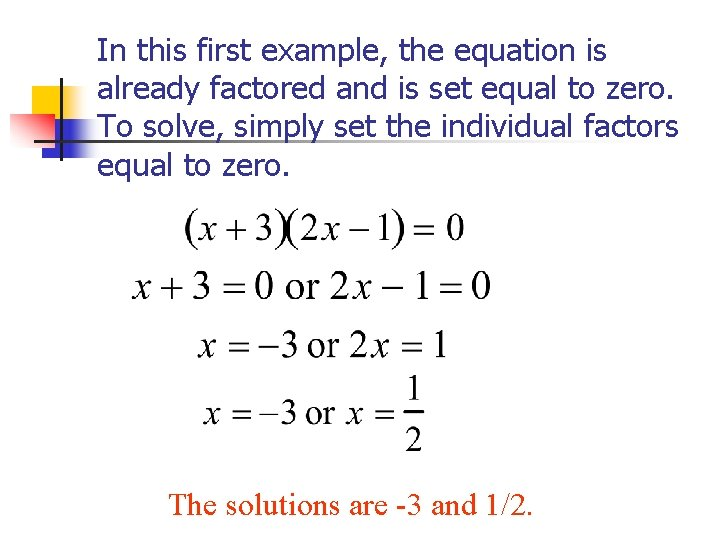In this first example, the equation is already factored and is set equal to zero. To solve, simply set the individual factors equal to zero. The solutions are -3 and 1/2.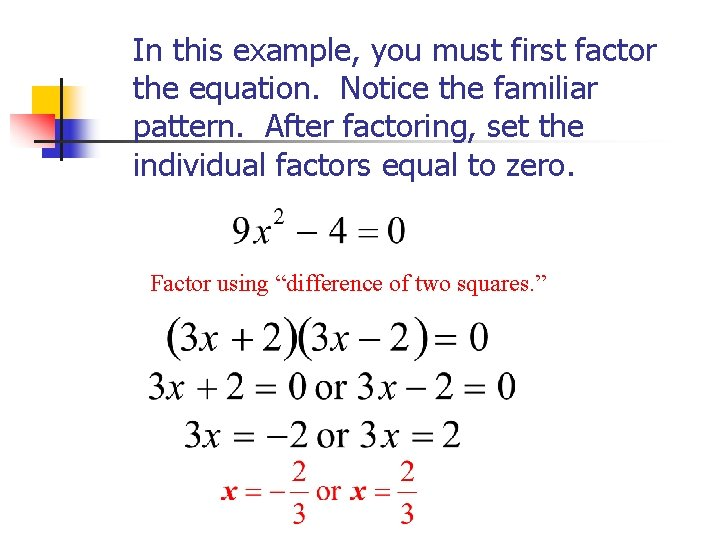In this example, you must first factor the equation. Notice the familiar pattern. After factoring, set the individual factors equal to zero. Factor using “difference of two squares. ”In the next example, you must set the equation equal to zero before factoring. Then set the individual factors equal to zero and solve.Re-write this example in the proper form. Notice that the leading coefficient is not one. Use an appropriate factoring technique. Then solve as you have done before.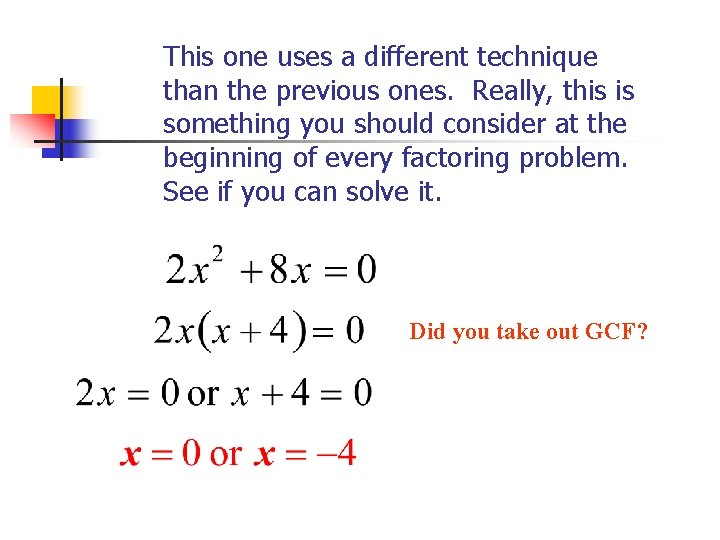This one uses a different technique than the previous ones. Really, this is something you should consider at the beginning of every factoring problem. See if you can solve it. Did you take out GCF?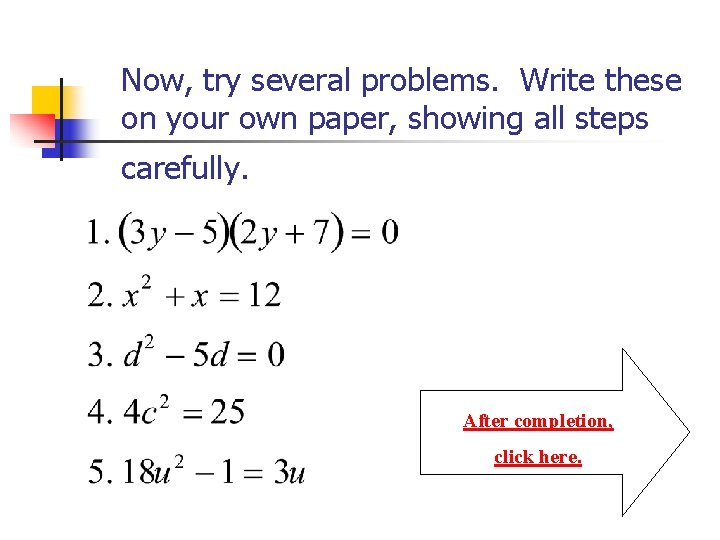Now, try several problems. Write these on your own paper, showing all steps carefully. After completion, click here.Here are the answers. For help, click on the numbers. n n n 1. 2. 3. 4. 5. If all are correct, you’re finished!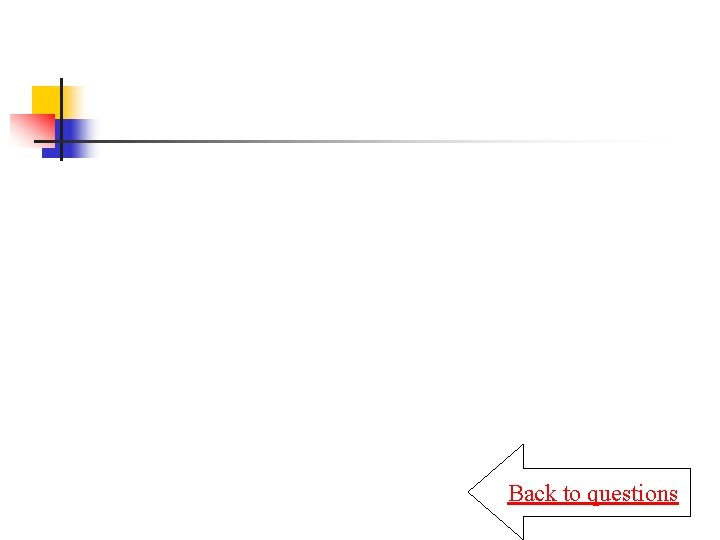Back to questions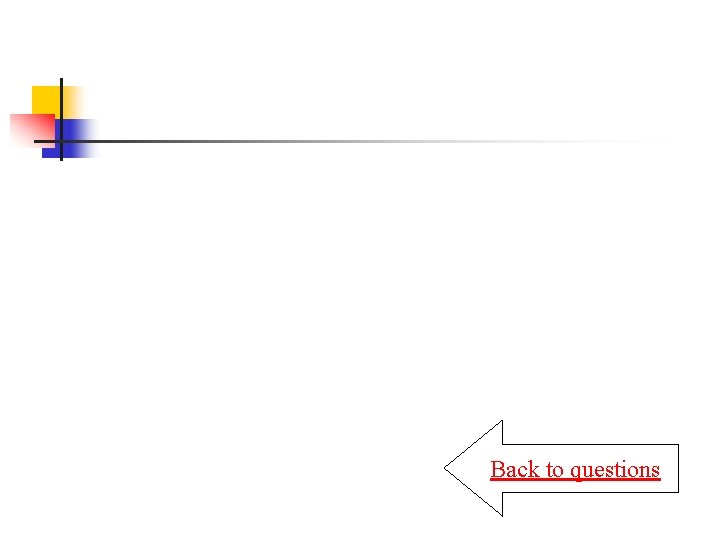Back to questions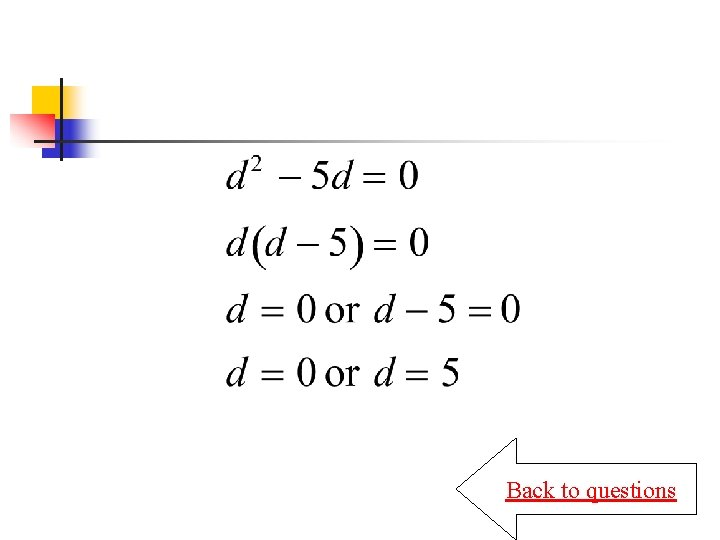Back to questions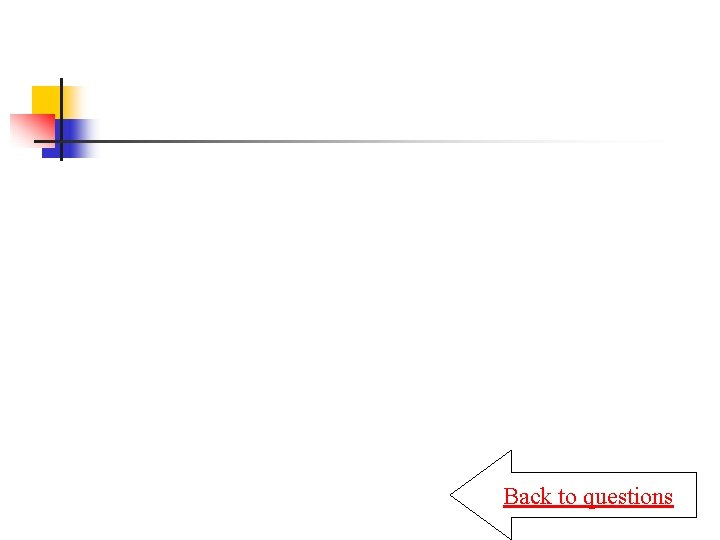Back to questionsBack to questions